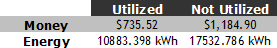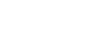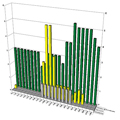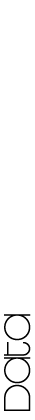The following charts summarize the data collected with stopwatches during the 6 one-hour observation periods throughout the week. We began data collection on Thursday, 8 March 2007 at 4:15 PM and finished Sunday, 11 March 2007 at 5:47 PM. Data from outside this time period would be relevant to our study, but it was not feasible to observe for 100% of the VRC’s business hours.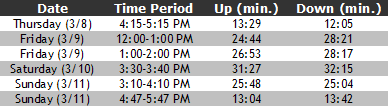We took these individual time measurements from both up and down escalators and converted the minutes into percentages of an hour to compare with our hypothesis. The chart below features the converted percentages.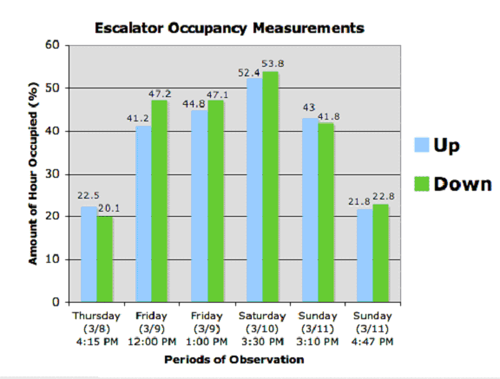The projected data shows that the escalator was utilized more than 50% of the time only during one observed hour period. To calculate our average disuse figure we added our collected data from each escalator and took the following averages: Down—38%, Up—38.6%, Average—38.3%. This figure shows that we met our hypothesis: the escalator was in use, overall, less than 50% of observed hours. To calculate the annual energy use of the escalator, we needed to size the motor for its energy output and used the following specifications.

Escalator Specifications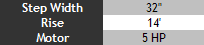(MEEB reference pg. 1472)

We converted the horsepower to 3.73 kW of energy output. To find the cost of energy output, we required the following energy prices.

Energy Prices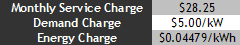(Commercial Energy Rate: http://www.eweb.org/news/projects/rates.htm)

The cost per hour for the motor would be \$0.178 with a monthly demand charge of \$18.65. To get a more accurate estimate of the actual energy use of the escalator, it was also necessary to calculate the cooling load that the escalator added to the Valley River HVAC system. We calculated there being an additional 3.73 kW of demand on the system, resulting an additional hourly charge of \$0.178.

Weekly Mall Hours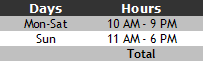(Mall Hours: http://www.valleyrivercenter.com/)

Using these hours, we calculated that it costs the mall \$27.448/week to operate the escalator based upon the motor and cooler output. This cost with demand charges over a year come to a total of \$1920.413/year and 28416.18 kWh/year to operate the escalator. We applied the escalator disuse figure calculated from our data (38.3%) to calculate the amount of resources appropriately utilized, that is, when people were using the escalator. The following is a breakdown of the annual energy use between times when the escalator is occupied and times it has zero-occupancy.

Escalator Resource Misappropriation